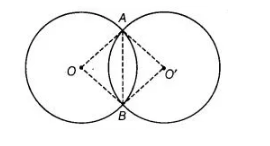# The congruent circles with centres O`
Question:

The congruent circles with centres O and O’ intersect at two points A and B. Then, ∠AOB = ∠AO’B.

Solution:

TrueJoin AB, OA  and OB, O’A and BO’.
In ΔAOB and ΔAO’B,

OA = AO’  [both circles have same radius]

OB = BO’ [both circles have same radius]

and AB= AB  [common chord]

ΔAOB = ΔAO’B   [by SSC congruence rule]

=> ∠AOB = ∠AO’B   [by CPCT]